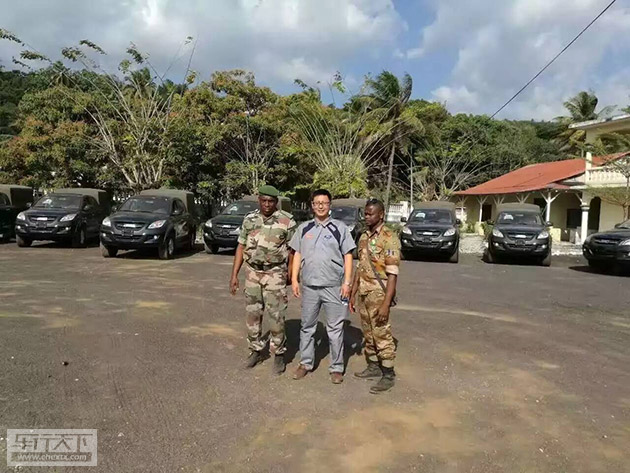## 靠谱灵动易改装 域虎皮卡军工品质闯世界

2017年01月13日 14:59 来源：www.chextx.com 超过：次关注

在解放军后勤部队服过役，

为联合国维和部队站过岗，

出席过反法西斯战争胜利70周年大阅兵，

见证了中非军援的国际友谊。

从中国军队到世界战场，

这辆皮卡都能从容展现自身实力，

它就是江铃域虎!

2017开年伊始，江铃域虎第5000台军车即将下线!域虎皮卡的军车品质是如何炼成的?让我们从去年9月的一次中非军援说起。科摩罗，阿拉伯语中的“月亮”，是非洲印度洋上一个风光秀美的岛国。科摩罗被伊兰的芳香熏陶着，阿拉伯的美学装点着，非洲的温暖环绕着，虽然是世界上最不发达的国家之一，却是对中国最友好的国家之一。在台湾、钓鱼岛、南海问题上，始终坚定无条件支持中国的立场;在5.12汶川大地震后，全国每年下半旗悼念中国四川地震遇难者; 在遇到华人时，会用“你好”微笑着打招呼。友好和尊重都是相互的，对于如此友善的非洲伙伴，中国也始终尽力提供援助。在这次中非军援培训中，域虎皮卡就作为中方的主要军援装备交付科方。之后，将正式成为科摩罗军队的制式装备。科摩罗总统阿扎利·阿苏玛尼在总统府接见我国工作组全体成员， 对中方一直以来对科摩罗军方的支持与帮助表示感谢。皮卡作为便宜高效的军用装备，一直是经济欠发达地区军队的标配。

皮卡能够受到军事用途的青睐得益于几点：首先是优秀的机动性，皮卡速度快、车身灵巧，可以“打一枪换一个地方”;其次是优秀的改装性能，一辆皮卡车可以根据战地需求，随时随地改装成雷达车，装甲车，随意加装导弹发射器、重机枪甚至是防空高射机枪等; 最后是出色的越野性能，皮卡作为非承载式车身车型，无论是在城市，还是在沙漠，行走起来都游刃有余。江铃域虎能够成为此次中非军援的装备，不仅是对其本身优异性能的认可，更是对自主品牌汽车在技术、质量、研发水平和外形设计等各方面的认可。

对于军事用途的车辆来说，可靠性一直是重中之重，谁也不希望在生死攸关的时刻，车辆出现任何问题。而江铃汽车作为国内皮卡行业的老牌企业，传承23年皮卡研发生产经验，又引入福特的全球化研发生产标准和优质供应链，在车辆性能可靠性方面是数一数二的，域虎作为其旗下的高端车型自然非常靠谱。此外，域虎作为众多皮卡爱好者改装的首选，其易改装、改装潜力大、改装后可靠性依然强悍的特点，也是其能够作为军备援助车型的关键。无论科摩罗军队是将其改装成医护车，还是改装为战斗车辆，都毫无障碍。军工品质奠定大国根基，世界的舞台展示中国实力。希望有越来越多的自主品牌能雄起，像这只“中国虎”一样，为中华民族的大国崛起贡献力量!

#### 相关文章

﻿
• 快速找车
• 选择品牌
• 选择品牌
• A  奥迪
• A  阿斯顿·马丁
• A  阿尔法·罗密欧
• B  宝沃
• B  布加迪
• B  巴博斯
• B  保时捷
• B  宾利
• B  奔驰
• B  宝马
• B  本田
• B  别克
• B  标致
• B  比亚迪
• B  宝骏
• B  北汽制造
• B  北汽新能源
• B  北汽幻速
• B  北汽威旺
• B  北京汽车
• B  奔腾
• B  北汽绅宝
• B  北汽昌河
• C  长安欧尚
• C  长安
• C  长安凯程
• C  长城
• D  大众
• D  道奇
• D  DS
• D  东南
• D  东风风神
• D  东风风行
• D  东风小康
• D  东风风度
• D  东风
• F  福特
• F  丰田
• F  菲亚特
• F  法拉利
• F  福田
• F  福迪
• F  福汽启腾
• G  观致
• G  广汽传祺
• G  广汽吉奥
• G  GMC
• H  红旗
• H  汉腾汽车
• H  哈弗
• H  哈飞
• H  海格
• H  海马
• H  华颂
• H  黄海
• H  华泰
• H  恒天
• J  几何汽车
• J  捷达
• J  吉利汽车
• J  捷豹
• J  Jeep
• J  江淮
• J  江铃
• J  金杯
• J  九龙
• J  金旅
• K  凯翼
• K  凯迪拉克
• K  克莱斯勒
• K  科尼塞克
• K  卡威
• K  开瑞
• L  路虎
• L  林肯
• L  劳斯莱斯
• L  兰博基尼
• L  雷克萨斯
• L  铃木
• L  领克
• L  雷诺
• L  理念
• L  力帆
• L  莲花汽车
• L  猎豹
• L  路特斯
• L  陆风
• M  马自达
• M  MG
• M  MINI
• M  玛莎拉蒂
• M  摩根
• M  迈凯轮
• N  纳智捷
• O  欧拉
• O  欧宝
• O  讴歌
• O  欧朗
• Q  奇瑞
• Q  起亚
• Q  启辰
• R  日产
• R  荣威
• R  瑞麒
• S  SERES赛力斯
• S  三菱
• S  斯威汽车
• S  萨博
• S  smart
• S  斯柯达
• S  斯巴鲁
• S  思铭
• S  双龙
• S  上汽大通
• S  双环
• T  特斯拉
• T  腾势
• W  蔚来
• W  沃尔沃
• W  WEY
• W  五菱汽车
• W  五十铃
• W  威兹曼
• W  威麟
• X  现代
• X  雪佛兰
• X  星途
• X  雪铁龙
• X  小鹏汽车
• X  西雅特
• Y  一汽
• Y  英菲尼迪
• Y  英致
• Y  依维柯
• Y  野马汽车
• Y  永源
• Z  众泰
• Z  中华
• Z  中兴
• Z  知豆
• 选择车系
• 选择车系
• 车型对比
• 选择品牌
• 选择品牌
• A  奥迪
• A  阿斯顿·马丁
• A  阿尔法·罗密欧
• B  宝沃
• B  布加迪
• B  巴博斯
• B  保时捷
• B  宾利
• B  奔驰
• B  宝马
• B  本田
• B  别克
• B  标致
• B  比亚迪
• B  宝骏
• B  北汽制造
• B  北汽新能源
• B  北汽幻速
• B  北汽威旺
• B  北京汽车
• B  奔腾
• B  北汽绅宝
• B  北汽昌河
• C  长安欧尚
• C  长安
• C  长安凯程
• C  长城
• D  大众
• D  道奇
• D  DS
• D  东南
• D  东风风神
• D  东风风行
• D  东风小康
• D  东风风度
• D  东风
• F  福特
• F  丰田
• F  菲亚特
• F  法拉利
• F  福田
• F  福迪
• F  福汽启腾
• G  观致
• G  广汽传祺
• G  广汽吉奥
• G  GMC
• H  红旗
• H  汉腾汽车
• H  哈弗
• H  哈飞
• H  海格
• H  海马
• H  华颂
• H  黄海
• H  华泰
• H  恒天
• J  几何汽车
• J  捷达
• J  吉利汽车
• J  捷豹
• J  Jeep
• J  江淮
• J  江铃
• J  金杯
• J  九龙
• J  金旅
• K  凯翼
• K  凯迪拉克
• K  克莱斯勒
• K  科尼塞克
• K  卡威
• K  开瑞
• L  路虎
• L  林肯
• L  劳斯莱斯
• L  兰博基尼
• L  雷克萨斯
• L  铃木
• L  领克
• L  雷诺
• L  理念
• L  力帆
• L  莲花汽车
• L  猎豹
• L  路特斯
• L  陆风
• M  马自达
• M  MG
• M  MINI
• M  玛莎拉蒂
• M  摩根
• M  迈凯轮
• N  纳智捷
• O  欧拉
• O  欧宝
• O  讴歌
• O  欧朗
• Q  奇瑞
• Q  起亚
• Q  启辰
• R  日产
• R  荣威
• R  瑞麒
• S  SERES赛力斯
• S  三菱
• S  斯威汽车
• S  萨博
• S  smart
• S  斯柯达
• S  斯巴鲁
• S  思铭
• S  双龙
• S  上汽大通
• S  双环
• T  特斯拉
• T  腾势
• W  蔚来
• W  沃尔沃
• W  WEY
• W  五菱汽车
• W  五十铃
• W  威兹曼
• W  威麟
• X  现代
• X  雪佛兰
• X  星途
• X  雪铁龙
• X  小鹏汽车
• X  西雅特
• Y  一汽
• Y  英菲尼迪
• Y  英致
• Y  依维柯
• Y  野马汽车
• Y  永源
• Z  众泰
• Z  中华
• Z  中兴
• Z  知豆
• 选择车系
• 选择车系
• 选择车型
• 选择车型
• 意见反馈